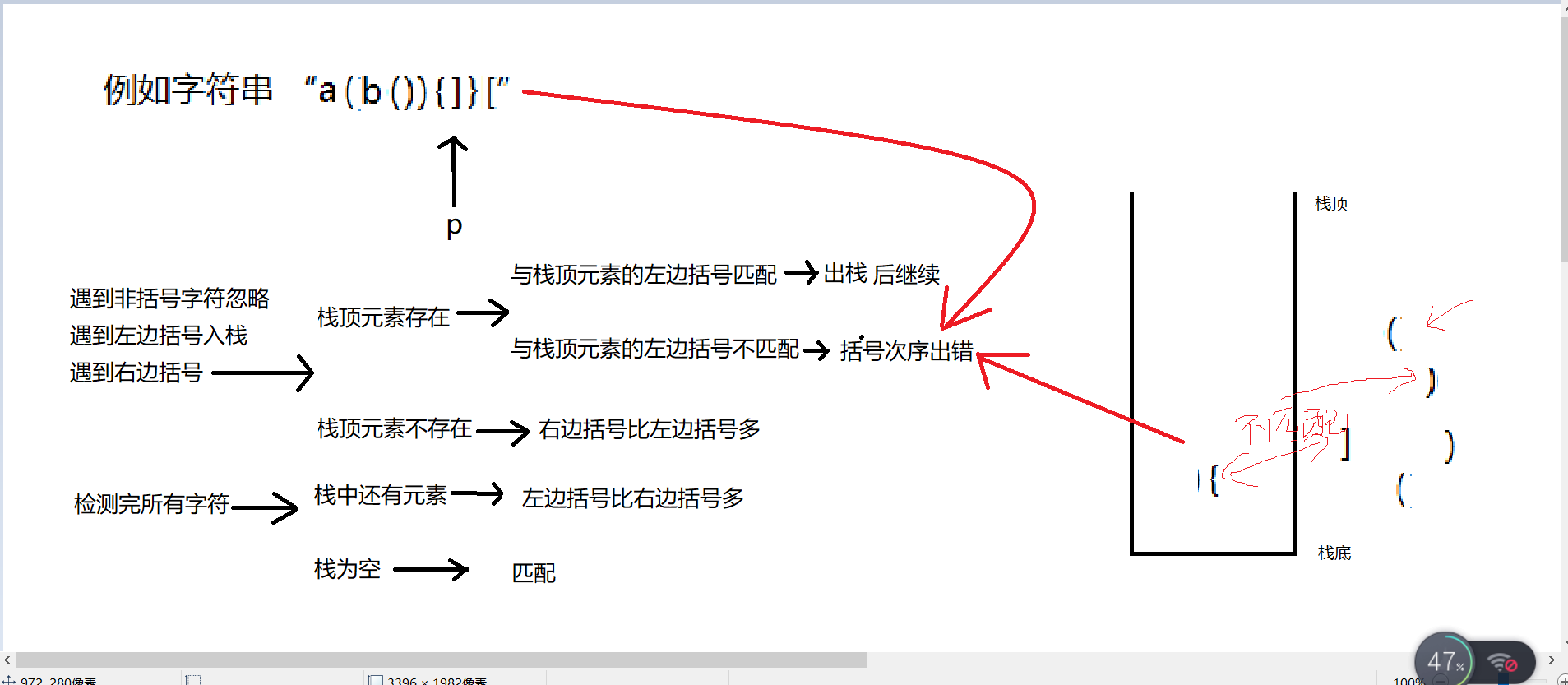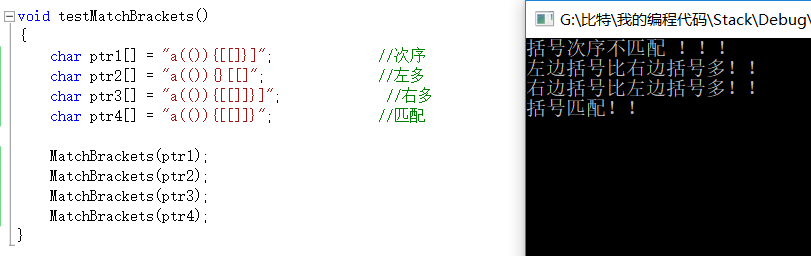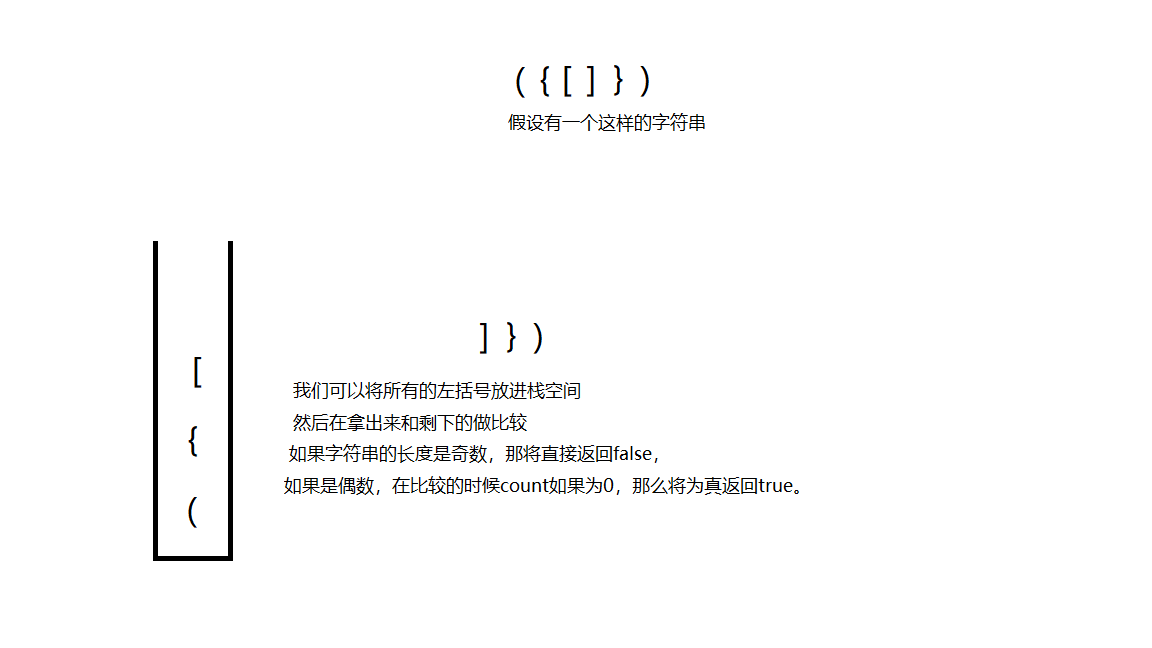• 来人！...一个字符串中有许多的括号！ 例如字符串 ptr1[] = “a(()){[[]}]”; //次序 ptr2[] = “a(()){}[[]”; //左多 ptr3[] = “a(()){[[]]}]”; //右多 ptr4[] = “a(()){[[]]}”; //匹...
来人！！上题目！
一个字符串中有许多的括号！ 例如字符串  ptr1[] = “a(()){[[]}]”; //次序  ptr2[] = “a(()){}[[]”; //左多  ptr3[] = “a(()){[[]]}]”; //右多  ptr4[] = “a(()){[[]]}”; //匹配  通过我们的眼睛，我们不难看出这些字符串的括号是否匹配，但是一旦字符串过长，便无法用眼睛了！！  于是乎，用一个函数、一串代码解决这个问题，不是特别煎蛋？？
恭迎大人阅读解题思路小心翼翼给大人递上代码
int Bracket(char ch)
{
if ('(' == ch || ')' == ch ||                         //判断该字符是否是括号字符
'[' == ch || ']' == ch ||
'{' == ch || '}' == ch)
return 1;
else
return 0;
}

//用栈检测括号是否匹配
void MatchBrackets(char* Ptr)
{
assert(Ptr);                                                                 //参数检测

int size = strlen(Ptr);                                                      //求字符串长度
int i = 0;
StackD s;                                                                    //创建栈

StackDInit(&s, size);                                                        //栈初始化，容量为size

if (size == 0)                                                             //字符串为空
{
printf("无括号，匹配！！ \n");
return;
}
else                                                                         //字符串不为空
{
for (; i < size; i++)                                                    //从头开始一次判断 每个字符
{
if (Bracket(Ptr[i]))                                                 //对字符进行判断，看其是否为括号字符
{
if ('(' == Ptr[i] || '[' == Ptr[i] || '{' == Ptr[i])             //字符为左边括号  入栈
StackDPush(&s, Ptr[i]);
else                                                             //字符是右边括号
{
if (StackDSize(&s) == 0)                                     //栈中没有左边括号，则说明右边括号多
{
printf("右边括号比左边括号多！！\n");
return;
}
else{                                                       //栈中有左边括号，判断是否匹配？  匹配则出栈一次，不匹配则说明括号次序不匹配！
char ch = StackDTop(&s);
if (ch == '(' && Ptr[i] == ')' ||
ch == '[' && Ptr[i] == ']' ||
ch == '{' && Ptr[i] == '}')
StackDPop(&s);
else
{
printf("括号次序不匹配 ！！！\n");
return;
}
}
}
}
}
if (StackDSize(&s) != 0)                                               //判断完所有字符之后，判断栈中是否还有左边括号！
{
printf("左边括号比右边括号多！！\n");
return;
}
}
printf("括号匹配！！\n");
}

证据在此！！！提醒：该函数调用了栈结构，关于栈结构的基本操作可以点这个链接  https://blog.csdn.net/code_zx/article/details/80812635  或者关注我，进主页查看哦展开全文数据结构
• #define MaxSize 50 //定义中元素最大个数 typedef struct{//结构体 ElemType data[MaxSize];//存放中元素 int top; //栈顶指针 }SqStack; //初始化 void InitStack(SqStack &S){ S.top=-1
啥也不多说直接上代码
#include <stdio.h>
typedef char ElemType;//将 ElemType声明成int类型

#define MaxSize 50  //定义栈中元素最大个数
typedef struct{//结构体
ElemType data[MaxSize];//存放栈中元素
int top;			   //栈顶指针
}SqStack;

//初始化栈
void InitStack(SqStack &S){
S.top=-1;
}

// 判断栈空
bool SatckEmpty(SqStack &S){
if(S.top==-1)
return true;//栈空
else
return false;//不空

}

//入栈
bool Push(SqStack &S,ElemType x){
if(S.top==MaxSize-1)//栈满报错
return false;
/**S.top=S.top+1;先指针加一
S.data[S.top]=x;再给指针指向的元素赋值**/
S.data[++S.top]=x;
return true;
}

//出栈
bool Pop(SqStack &S,ElemType &x){
if(S.top==-1)//栈空报错
return false;
x=S.data[S.top];//先取出栈顶元素
S.top=S.top-1;//栈顶指针减一
return true;
}

bool breaketCheck(char str[],int length){
SqStack S;
InitStack(S);//初始化一个栈
for(int i=0;i<=length;i++){
if(str[i]=='(' || str[i]=='{' || str[i]=='['){
Push(S,str[i]);//扫描到左括号都入栈
}else{

char topElem;
Pop(S,topElem);//取出栈顶元素和右括号匹配
if(str[i]==')' && topElem!='(')
return false;
if(str[i]=='}' && topElem!='{')
return false;
if(str[i]==']' && topElem!='[')
return false;
}

}
return SatckEmpty(S);//若最后的栈是空的则匹配成功

}

int main(){

char a;
int length=10;
printf("请输入5对括号\n");
for(int i=0;i<=10;i++)
{
scanf("%c",&a[i]);
}
if(breaketCheck(a,length)){
printf("括号匹配成功\n");
}else{
printf("括号匹配失败\n");
}
for(int i=0;i<=10;i++)
{
printf("%c\n",a[i]);
}

}


运行结果
请输入5对括号
[]{([])[]}
括号匹配成功
[
]
{
(
[
]
)
[
]
}

展开全文数据结构
• 括号必须用相同类型的右括号闭合。 左括号必须以正确的顺序闭合。 注意空字符串可被认为是有效字符串。 示例 1: 输入: “()” 输出: true 示例 2: 输入: “()[]{}” 输出: true 示例 3: 输入: “(]” 输出: false...
给定一个只包括 ‘(’，’)’，’{’，’}’，’[’，’]’ 的字符串，判断字符串是否有效。
有效字符串需满足：
左括号必须用相同类型的右括号闭合。
左括号必须以正确的顺序闭合。

注意空字符串可被认为是有效字符串。 示例 1:
输入: “()” 输出: true
示例 2:
输入: “()[]{}” 输出: true
示例 3:
输入: “(]” 输出: false
示例 4:
输入: “([)]” 输出: false
示例 5:
输入: “{[]}” 输出: true首先是第一个代码，创建出一个长度为字符串大小一半的空间，如果有左括号的话，将所有的左括号放进空间，然后在拿出来作比较，这个 代码的缺陷是 没有释放申请的空间，可能会导致内存泄漏问题，下面附上代码。
bool isValid(char * s)
{
int length = 0;
while(*(s+length))
{
length++;  //获取字符串长度
}
char *ptr = (char*)malloc(sizeof(char)+length/2+1);//这里申请的空间是比源字符串长度多了两个空间，因为如果源字符串的长度是三个的话，在下面的for循环中就越界了，而且a++在前，新的字符串中开始储存的地址是ptr,所以就多给了两个空间。
memset(ptr,0,length/2);//初始化
int i, a = 0;
for(i = 0; i < length; i++)
{
if((*(s+i) == '(') ||( *(s+i) == '{') || (*(s+i) == '['))
{
a++;
*(ptr+a) = *(s+i);

}
//'(' 和‘)’的ASCII码值差1，‘{’ 和‘}’，‘[’ 和 ']'差2
else if((*(s+i) == (*(ptr+a)+1))||(*(s+i) == (*(ptr+a)+2)))
{
a--;
}
else
return 0;
}
if(a)
return 0;
return 1;
}


然后下面是一个改良版本，申请的空间是和源字符串相同的大小，防止出现全部都是左括号的情况，并且解决了预防了内存泄漏的问题
bool isValid(char * s){
int len = strlen(s);
if(len % 2 == 1) // 奇数直接返回
return false;
char *temp = (char*)malloc(len);
//考虑‘（（（’的情况
memset(temp, 0, len);
int i, count = 0;
for(i = 0; i < len; i++)
{
if(s[i] == '('||s[i] == '{'||s[i] == '[')
{
temp[count] = s[i];
count++;
}
else if((count != 0)&&((s[i] == temp[count-1]+1)||(s[i] == temp[count-1] + 2))）
{
count--;
}
else
{
free(temp);//将申请的空间释放
return false;
}
}
return temp;
if(count == 0)
return true;
return false;
}


展开全文• 利用括号匹配算法 C语言数据结构 利用括号匹配算法 C语言数据结构
• #用来解决括号匹配问题 挺简单的一道题，结果因为自己在写Push进行赋值的时候多写了一道等号，导致压入栈时出现乱码，我去！代码如下： #include&amp;lt;stdio.h&amp;gt; #include&amp;lt;stdlib.h...
#用栈来解决括号匹配问题 挺简单的一道题，结果因为自己在写Push进行赋值的时候多写了一道等号，导致压入栈时出现乱码，我去！代码如下：
#include<stdio.h>
#include<stdlib.h>
#include<string.h>
#define Maxsize 50
typedef char Datatype;
typedef struct{
Datatype data[Maxsize];
int top;
}SqStack;
void InitStack(SqStack *S)
{
S->top=-1;
}
int StackEmpty(SqStack *S)
{
if(S->top==-1)
return 0;
else
return 1;
}
int Push (SqStack *S,Datatype x)
{
if(S->top==Maxsize-1)
return 0;
else
S->data[++(S->top)]=x;
return 1;
}
int Pop(SqStack *S, Datatype *x)
{
if(S->top==-1)
return 0;
else
*x=S->data[(S->top)--];
return 1;
}
int GetTop(SqStack S,Datatype *x)
{
if(S.top==-1)
return 0;
else
*x=S.data[S.top];
return 1;
}
int Bracketscheck(SqStack *S,char *str)
{
InitStack(S);
char e;
int i=0;
while(str[i]!='\0')
{
switch(str[i]){
case '(':
Push(S,'(');
break;
case '{':
Push(S,'{');
break;
case '[':
Push(S,'[');
break;
case ')':
Pop(S,&e);
if(e!='(')
return 0;
break;
case '}':
Pop(S,&e);
if(e!='{')
return 0;
break;
case ']':
Pop(S,&e);
if(e!='[')
return 0;
break;
default:
break;

}
i++;
}
int h=StackEmpty(S);
if(h==1)
return 0;
else
return 1;
}
int main()
{    SqStack S;
char str[Maxsize];
printf("请输入你要收入的字符串：");
scanf("%s",str);
int h=Bracketscheck(&S,str);
if(h==0)
printf("括号不匹配");
else
printf("括号匹配");
return 0;
}


展开全文• 算法思路 使用一个字符串数组保存括号式。...// 括号匹配 _Bool parChecker() { int length, i; // 定义括号匹配长度 LiStack L; L = initStack(); // 初始化; char symbolString; // 使用char数据结构 算法
• 问题描述】 在某个字符串（长度不超过100）中有左、右括号和大小写字母；规定任何一个左括号都从内到外与在它右边且距离最近的有括号匹配。...括号匹配问题是堆栈的典型应用之一，每一个右括号必定与其之前未被...堆栈 字符串 数据结构
• 现在，有一行括号序列，请你检查这行括号是否配对。 输入数据保证只含有"[","]","(",")"四种字符输出如果该字符串中所含的括号是配对的，则输出Yes,如果不配对则输出No 代码如下 #include #...java IDEA
• 条件 给定一个只包括 '('，')'，'{'，'}'，'['，']' 的字符串 s ，判断字符串是否有效。... printf("请输入要匹配括号组："); fflush(stdout);//清空输出缓冲区（否则我上面打印不出来） scanf("%s", str); int le数据结构
• 实现括号匹配：依次扫描所有字符，遇到左括号则入栈，遇到右括号则弹出栈顶元素检查是否匹配。 匹配失败情况： 1、左括号单身 2、右括号单身 3、左右括号不匹配 代码： //用顺序实现括号匹配 #include<...数据结构
• printf("右括号比左括号多"); return; } else { GetTop(S,x); if(x=='{'&&a[i]=='}'||x=='['&&a[i]==']'||x=='('&&a[i]==')') { Pop(S,x); Pop(S,x); ...
• ## C语言用栈实现括号匹配问题

千次阅读 多人点赞 2020-03-09 12:17:29
例如：{}[()]、{[()]}、()[]{}这种大中小括号成对出现（位置不限）则为括号匹配，反之则不匹配，如{()[ 接下来看一下实现方式 的定义以及相关操作 //的定义 typedef struct{ char elem[stack_size]; int top;...
• 基本特点：“后进先出”，好比子弹上膛，最后放入弹夹中的子弹最先被射出，正因为有此特性，可以解决许多实际问题，例如即将讨论的括号匹配问题。 多说不易，来张图唤醒一下。 子弹上膛示意图 的特性类似于...数据结构 算法
• 输入一包含（和）的字符串，检测括号是否匹配(其中括号能嵌套括号），并输出括号是否匹配的信息（匹配，缺少左括号，缺少右括号）。
• 我们在编写程序的时候经常会遇到括号不匹配而导致语法错误的问题，现在我们可以通过来自己实现括号匹配问题，了解其实现细节。 我们假设在表达式中只有()[]这两种括号，其嵌套的方式是随意的。检验括号是否匹配...
• 二、方便起见，这里我们使用的顺序存储结构来存储待匹配的元素： 因为存储的是括号，所以数据部分是char类型。 我们开辟了10个连续的char型空间存储元素，1个Int型空间存储指针，指针的值为数组下标。 #include<...
• ## C语言括号匹配问题

千次阅读 2019-03-06 15:27:10
功能：可进行括号匹配，输入可包括{}, [], (), 其他任意字符 输入示例： 3 {{{{{{{[[[[]]]]}}}}}}} {{{{{[[[(())]}}}}} ((({{{{{[]}}}}}))) 输出示例： Yes No Yes Auth...
• 宁是否发现了不舒服的地方，oh这种事是无法让强迫症忍受的，没错，这里的括号是不匹配的，就像C语言中，如果你输入的括号不能匹配，那么编译就会出错，如何检测括号是否匹配就是我们今天的任务。 先谈谈思路吧，首先...
• ## 栈的应用之括号匹配（c语言）

千次阅读 多人点赞 2018-10-07 16:38:40
#include &amp;amp;amp;lt;stdio.h&amp;amp;amp;gt; #include &...//***的应用之括号匹配***/ typedef struct { char data[MAXSIZE]; int top; }*sqStack,sepStack; sqStack init数据结构
• 运用的知识当输入的字符串扫瞄时遇到左括号就进栈，右括号就弹。最后查看括号是否匹配压栈出栈
• C语言括号匹配问题解决方法，给定一个输入的字符串，包含括号（和），能够找出（和）的个数，并指出（或者）不正确的位置。
• 2、假设一个算术表达式中可以包含三种括号：园括号“(”和“)”、方括号“[”和“]”、花括号“{”和“}”，且这三种括号可按任意的次序嵌套使用。编写判别给定表达式中所含括号是否正确配对出现的算法（已知表达式...
• printf("括号匹配失败：右括号比左括号多！"); return FALSE; } if(s[i]==')')//检查()匹配 { if (ch == '(') { Pop(&stack); continue; } else { printf(...
• 问题描述 现在有一行括号序列，请你检查这行括号是否配对。 输入说明 第一行输入一个数N（0<N<=100）,表示有N组测试数据。后面的N行输入多组输入数据，每组输入数据都是一个字符串S(S的长度小于10000，且S...数据结构
• 题目描述现在，有一行括号序列，里面只包含”(“,”)”,”[“,”]”四种符号，请你检查这行括号是否配对。 如： []是匹配的 ([])[]是匹配的 ...定义一个计数器用来判断正反括号的数量，遇见 { 则count+1
• 实现括号匹配： 依次扫描所有字符，遇到左括号入栈，遇到右括号则弹出栈顶元素检查是否匹配。 匹配失败的情况： 左括号单身 右括号单身 左右括号不匹配 二、例题 给定一个只包括 '('，')'，'{'，'}'，'['，']'...数据结构
• 1、C语言栈括号匹配算法 一起加油兄弟姐妹们！！！冲冲冲 /** * 思想很简单就是左括号进栈，遇到右括号的时候，就把栈顶元素取出，然后匹配，然后对结果进行不用的处理 * 括号匹配算法： * 匹配失败的可能： ...算法 数据结构
• c语言实现括号匹配的检验问题 #include<stdio.h> #define maxsize 8 int match() { int stack[maxsize]; int top=-1; char str; gets(str); int i=0; while(str[i]!=’\0’) { if(str[i]=’(’ || str[i]......

# 括号匹配问题栈c语言c语言 订阅